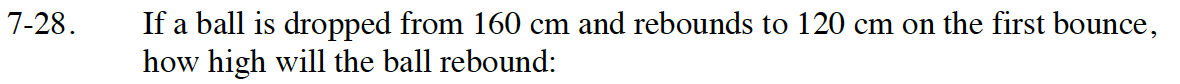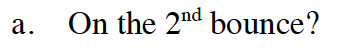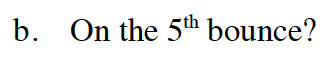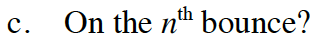Home > CC4 > Chapter 7 > Lesson 7.1.2 > Problem7-28

7-28.
1. If a ball is dropped from 160 cm and rebounds to 120 cm on the first bounce, how high will the ball rebound: Homework Help ✎

1. On the 2nd bounce?

2. On the 5th bounce?

3. On the nth bounce?What is the ratio between the rebound height and the original height?

$\text{The rebound ratio is }\frac{120 \text{ cm}}{160 \text{ cm}}=0.75.$

≈ 37.97 cmt(n) = 160(0.75)n